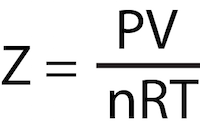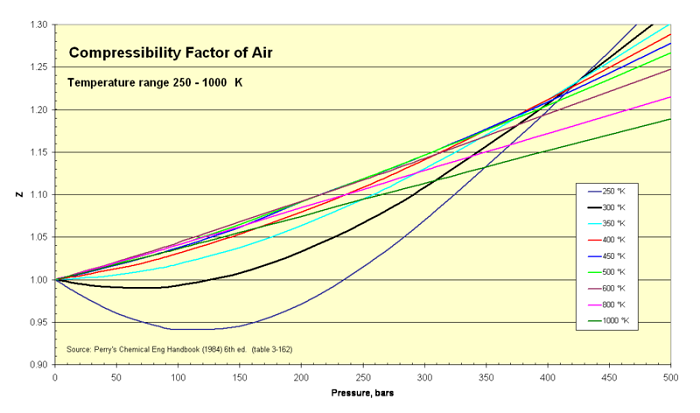# Gas Compressibility Factor

Instructor: Amy Lange

Amy has taught university-level earth science courses and has a PhD in Geology.

The ideal gas law describes how gases vary with temperature and pressure, but most gases exhibit non-ideal behavior. This gas compressibility factor is used to quantify this difference between real and ideal gases.

## Ideal Gases

Sometimes in order to understand a topic, we must first learn a simplified version and then learn exceptions and variations to this rule. When you first start learning about the behavior of gases, you were taught the ideal gas law and how to solve equations using this law. The ideal gas law describes how gases behave with changing pressure, volume, and temperature. The equation is PV = nRT, where P is pressure, V is volume, n is the number of moles, R is the ideal gas constant and T is temperature.

However, you'll notice by the name that this is the ideal gas law. What happens if a gas doesn't behave 'ideally?' Turns out that non-ideal, or real gases, will behave in ways that differ from the relationship described in the ideal gas law. We can quantify this difference using the gas compressibility factor, represented by the variable Z. You can also think of the gas compressibility factor as the ratio of the molar volume of the gas compared to the molar volume of the ideal gas at the same conditions.

## Gas Compressibility Factor Equation

The equation for the gas compressibility factor is:where Z is the gas compressibility factor, P is pressure, V is volume, n is the number of moles, R is ideal gas constant and T is temperature.

If you have an ideal gas, Z will be 1. Because remember, the ideal gas law states that PV = nRT, so a ratio of PV/nRT would be one because PV and nRT equal each other. For real gases, this number is not 1 and the deviation can be either positive, Z > 1, or negative, Z < 1.

## Understanding the Gas Compressibility Factor

You may wonder why real gases will deviate from the ideal gas law. Gases can exhibit non-ideal behavior due to their internal atomic interactions, such as attraction or repulsion forces during collisions between atoms. However, it's more common to have non-ideal behavior in extreme conditions like high temperature or high pressure. Thankfully, the ideal gas law is a close enough approximation to use most of the time. However, we're going to look at an example of how air displays non-ideal behavior in some situations.

The air we breath is composed of approximately 78% nitrogen, 21% oxygen, and 1% argon. It also has a pretty good approximation of ideal gas behavior, as we'll see in the following graph.This graph shows the Z factor of air at varying pressures and temperatures. The temperature shown here is in Kelvin. Remember that 273 K = 0 degrees Celsius = 32 degrees Fahrenheit. So the temperatures here range from -10 degrees Fahrenheit to over 1300 degrees Fahrenheit! For pressure, 1 bar is approximately the atmospheric pressure at sea level, so this can be considered a standard pressure.

To unlock this lesson you must be a Study.com Member.

### Register to view this lesson

Are you a student or a teacher?

### Unlock Your Education

#### See for yourself why 30 million people use Study.com

##### Become a Study.com member and start learning now.
Back
What teachers are saying about Study.com

### Earning College Credit

Did you know… We have over 160 college courses that prepare you to earn credit by exam that is accepted by over 1,500 colleges and universities. You can test out of the first two years of college and save thousands off your degree. Anyone can earn credit-by-exam regardless of age or education level.